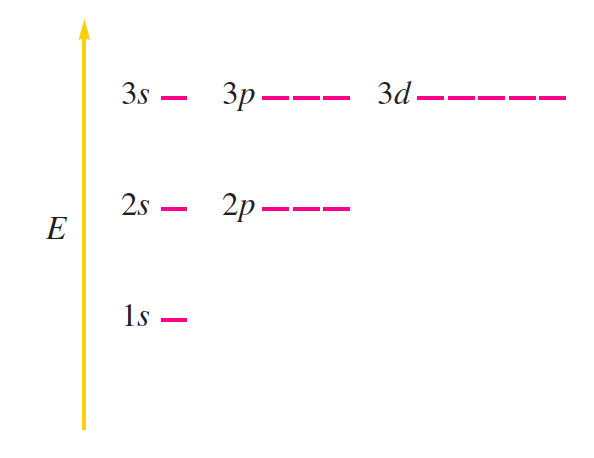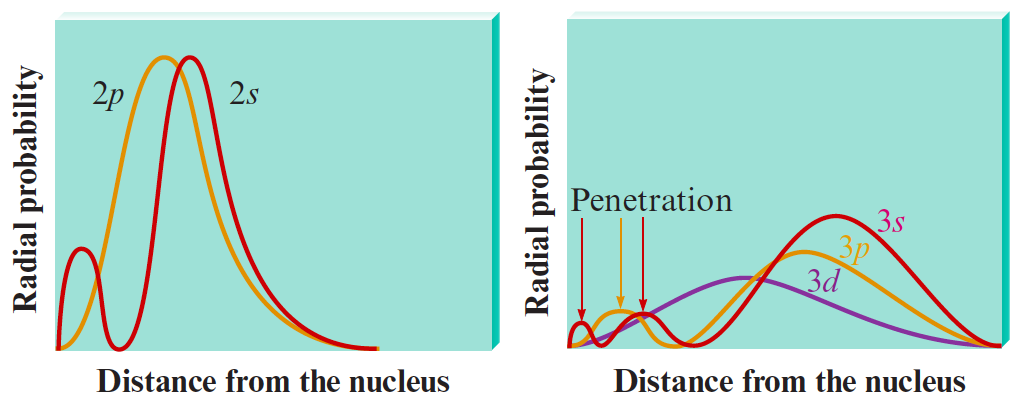# Problem: The relative orbital levels for the hydrogen atom can be represented asDraw the relative orbital energy levels for atoms with more than one electron and explain your answer. Also explain how the following radial probability distributions support your answer.

⚠️Our tutors found the solution shown to be helpful for the problem you're searching for. We don't have the exact solution yet.

###### Problem Details

The relative orbital levels for the hydrogen atom can be represented asDraw the relative orbital energy levels for atoms with more than one electron and explain your answer. Also explain how the following radial probability distributions support your answer.